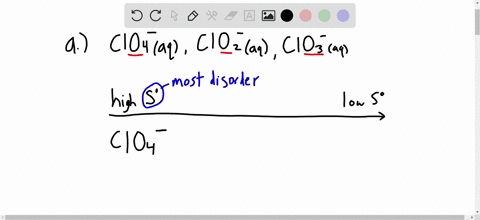Enroll in one of our FREE online STEM summer camps. Space is limited so join now!View Summer Courses### Without consulting Appendix $\mathrm{B}$ , arrang…

06:17University of Massachusetts - Lowell

Need more help? Fill out this quick form to get professional live tutoring.

Get live tutoring
Problem 27

Without consulting Appendix $\mathrm{B}$ , arrange each group in order of increasing standard molar entropy $\left(S^{\circ}\right) .$ Explain.
(a) Glucose $\left(\mathrm{C}_{6} \mathrm{H}_{12} \mathrm{O}_{6}\right),$ sucrose $\left(\mathrm{C}_{12} \mathrm{H}_{22} \mathrm{O}_{11}\right),$ ribose $\left(\mathrm{C}_{5} \mathrm{H}_{10} \mathrm{O}_{5}\right)$
(b) $\mathrm{CaCO}_{3}, \mathrm{Ca}+\mathrm{C}+\frac{3}{2} \mathrm{O}_{2}, \mathrm{CaO}+\mathrm{CO}_{2}$
(c) $\mathrm{SF}_{6}(g), \mathrm{SF}_{4}(g), \mathrm{S}_{2} \mathrm{F}_{10}(g)$

Check back soon!

## Discussion

You must be signed in to discuss.

## Video Transcript

So Promise 27 as says, to sort by increase in, start them different combinations of molecules by increasing standard molar entropy. So first off in a, we have glucose, sucrose and ribose, and we have the respective, um, formulas there as well. So what you notice is that if you look at the formulas, you'll have a different, um in So sucrose has the highest amount of Adams in their rivals has the least amount of Adams. So I guess you can see that. So with entropy, um, as the amount of stuff increases amount of interest, entropy or standard molar, entropy also increases as well. And in this guest states to really matter because we're just comparing, uh, the the molar mass and molecular formula. They don't even give a state. So that's just how it is anyways. So now we have the least amount of molecules over here with rebels and the highest men here side Not highest Hi smaller mass. Sorry, Lois, with O. R. Moeller Mass highest Mueller mess. And we have a glucose in the middle here, So let's focus on rivals and sue crops, and we noticed that you have C 12 2111 any of C five h 10 05 That is both, uh, this is the smallest at all. The mess they said. And as you as you decreased the amount of Adams he else have less electrons. That's combinations. That's bonds less variability. So let's have this as the least entropy. And this is the highest in tribute. And screw cocoas goes in the middle there. Okay, So for the sake of explanations, let's give to see because C is very similar to a So we have silver hexafluoride, so protection for right or? And I saw a deck of fluoride kind of mouth eso like, um a we have the smallest muller mass, which is s f four here. So let's that at the bottom, cause that has a lower entropy, somewhat less animals. Lesson like transfers, variability, less bonds, least entropy. Okay. And then we have the s two f 10. I'm just gonna call it that for now. And as this is diess two silver sand 10 florins, there's more. Like I said, more Adams were bonds, more variability, more electrons to less at the highest and suffers except for is soon sitting comfortably in the middle here. Okay, so between sea and a the name of the game is Moeller mass. Essentially a smaller mass increases standard mole entry, tense toe also increase. Assuming the species, they're all the same. Okay. And now we have B b is different from a and sees, which is why I'm doing this one differently from the other two. So we have calcium carbonate here. Sorry, cousin carbonate, calcium, carbon and 3.5 moles of oxygen. And we have cause him outside and carbon dioxide. So, uh, that's sort of like this. This thing of like, this casting carpet is like a huh Lego set or a like a piece, But it's all assemble together. You have three Lego bricks here. That's do this. Ah, uh, yes. Here, here and we have here. Okay, let's just say calcium urge costumes. Black carbon is red and oxygen is blue. Okay, Jessica, nor the count. For now, we just want to get the idea. So we have calcium carbonate here we have our Lego bricks are fully assembled. Michael Brick. Right now let's go to calcium oxide and calcium dye Akhtar sequestering carbon dioxide. And for the sake of argument. Let's say there's an auction attached to here and is the Mr Carbon and the oxygen. Okay, we a sweet we ripped apart our, um are leg was set and now there's they're in two different places. There's so much more variability in that kiss and for the calcium, carbon and oxygen. So we have We're splitting this part as we're splitting this piece of part as well. So now we have three pieces floating around, not exactly interacting with each other early. Could be. But I saw the point here. What the point is is that they're all rent by, says too much higher entry state. Okay, so with that in mind, let's have our lowest entropy state or um, species with lowest entropy is the casting prep innate. Next will be the two Lego bricks. These air to several, like bricks, are separated. Think of Rex is the calcium oxide and carbon dioxide. And next, we're for three Lego bricks floating around. We have the calcium, carbon and the oxygen. Okay,